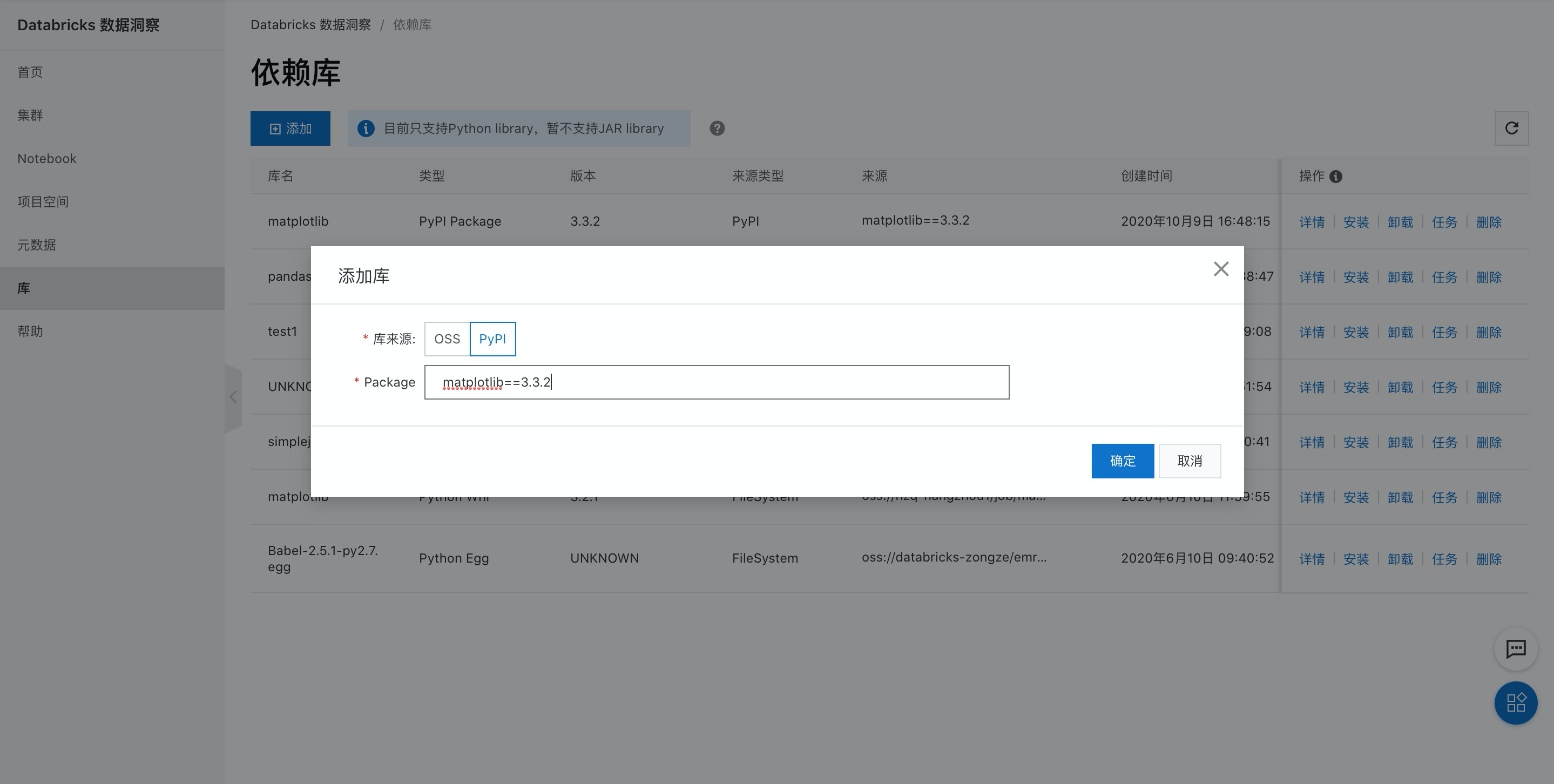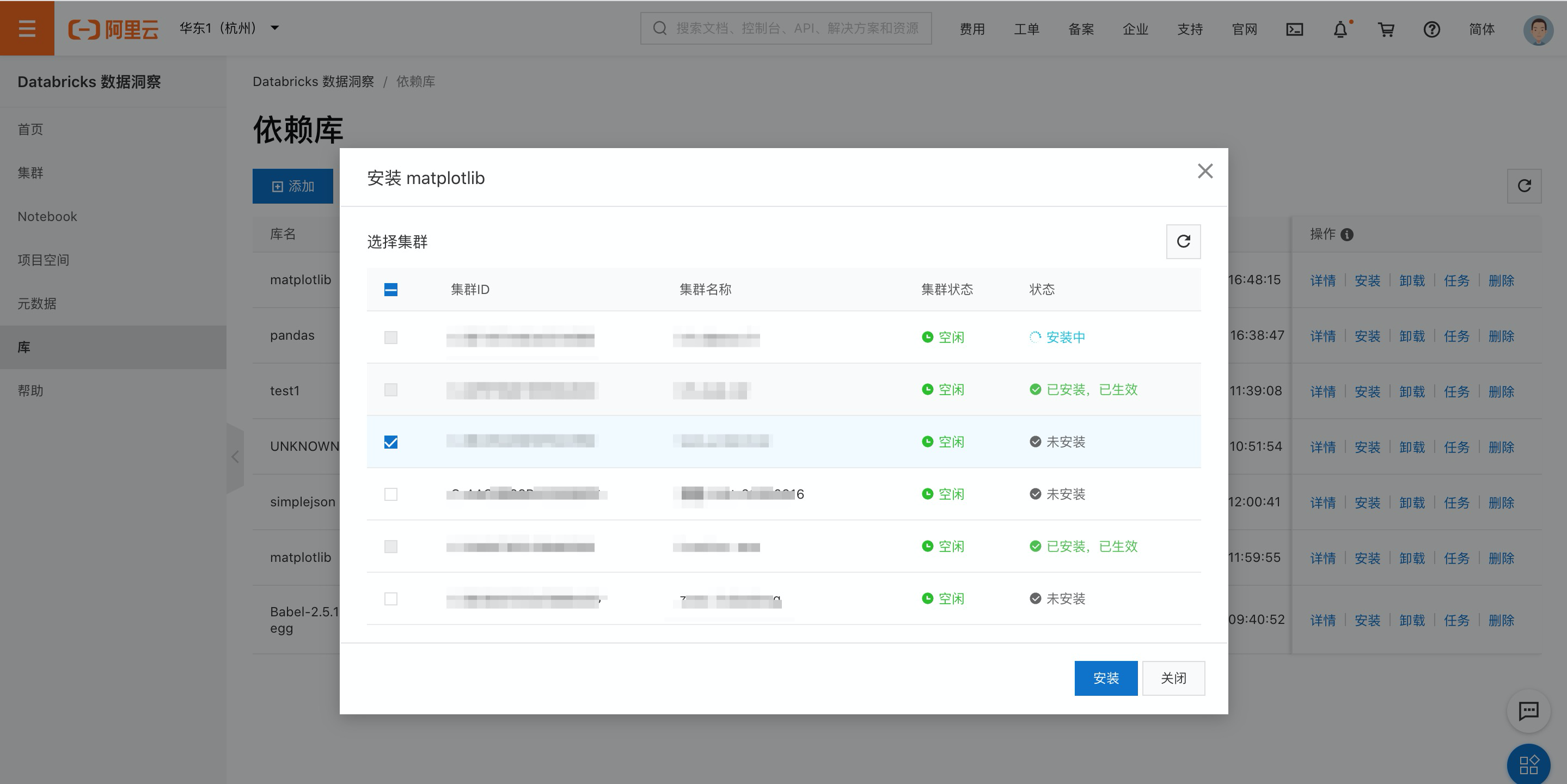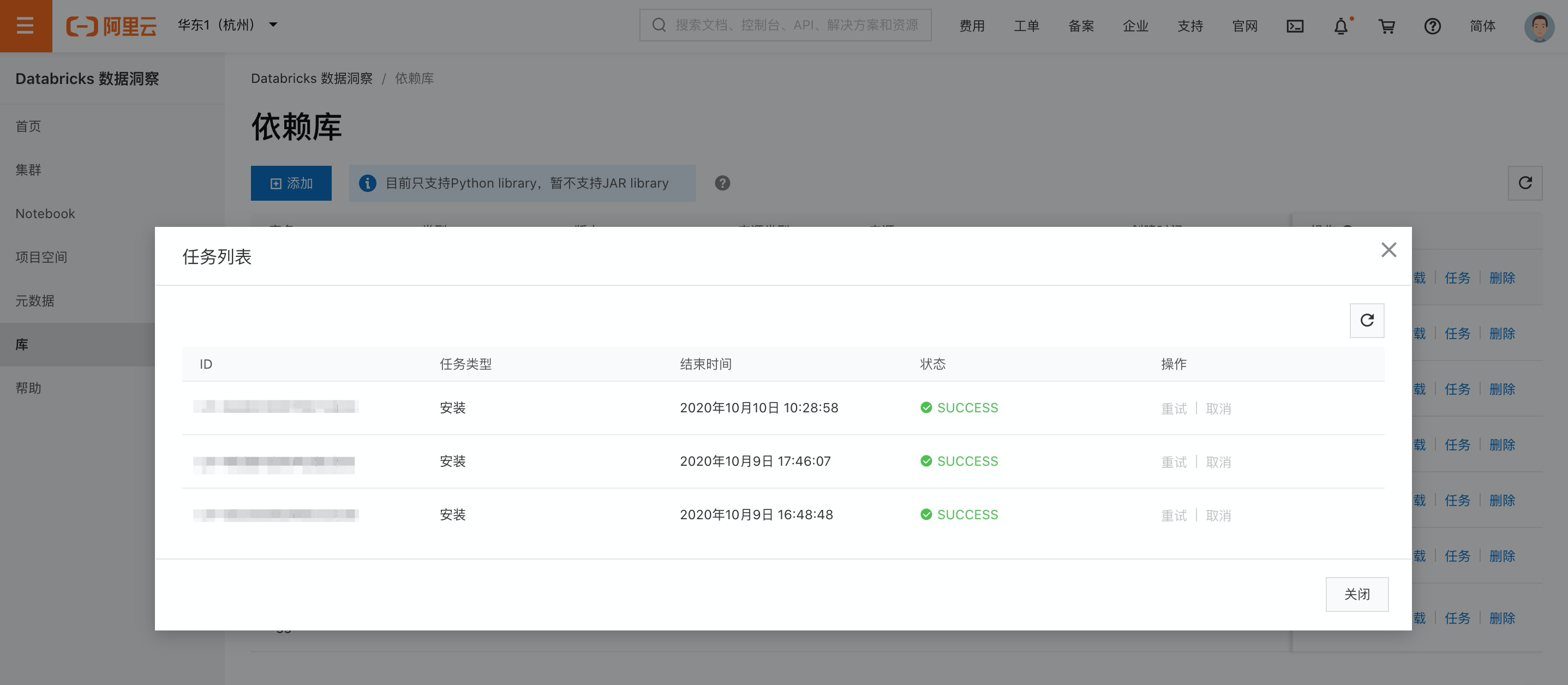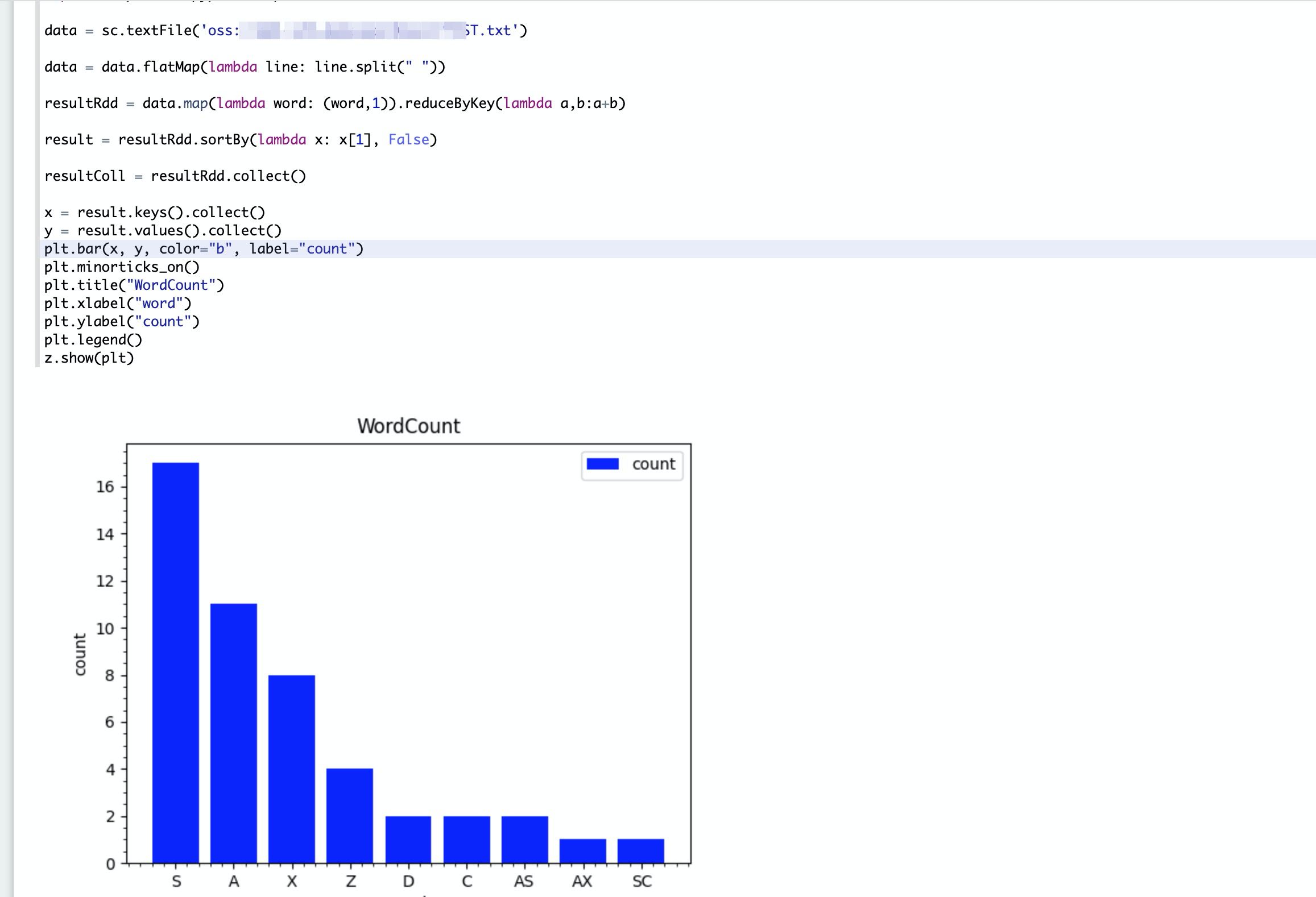# DDI PySpark示例及库的使用

## 前提条件

• 已注册阿里云账号，详情请参见阿里云账号注册流程

• 已开通 Databricks数据洞察服务。

• 已完成云账号的授权，详情请参见角色授权

• 已使用OSS管理控制台创建非系统目录存储空间，详情请参见创建存储空间

警告

首次使用DDI产品创建的Bucket为系统目录Bucket，不建议存放数据，您需要再创建一个Bucket来读写数据。

说明

DDI支持免密访问OSS路径，结构为：oss://BucketName/Object

• BucketName为您的存储空间名称；

• Object为上传到OSS上的文件的访问路径。

例：读取在存储空间名称为databricks-demo-hangzhou文件路径为demo/The_Sorrows_of_Young_Werther.txt的文件

``````// 从oss地址读取文本文档
val text = sc.textFile("oss://databricks-demo-hangzhou/demo/The_Sorrows_of_Young_Werther.txt")``````

## 步骤一：创建Databricks 数据洞察集群

• 创建Databricks 数据洞察集群，详情参见集群创建

## 步骤二：添加依赖库并安装

• 添加matplotlib库，本示例使用PyPI方式添加，详情参见管理库• 单击安装按钮，安装依赖到开发集群。• 等待安装完成，可单击任务按钮查看。## 步骤四：数据开发

• Notebook使用，详情参见Notebook使用

• 数据开发，将以下代码写入note文件，如下图所示。

``````%pyspark
import matplotlib.pyplot as plt

data = sc.textFile('oss://xxx/xxx/TEST.txt')

data = data.flatMap(lambda line: line.split(" "))

resultRdd = data.map(lambda word: (word,1)).reduceByKey(lambda a,b:a+b)

result = resultRdd.sortBy(lambda x: x, False)

resultColl = resultRdd.collect()

x = result.keys().collect()
y = result.values().collect()
plt.bar(x, y, color="b", label="count")
plt.minorticks_on()
plt.title("WordCount")
plt.xlabel("word")
plt.ylabel("count")
plt.legend()
z.show(plt)``````
• 单击右上角运行按钮，等待任务结束查看结果